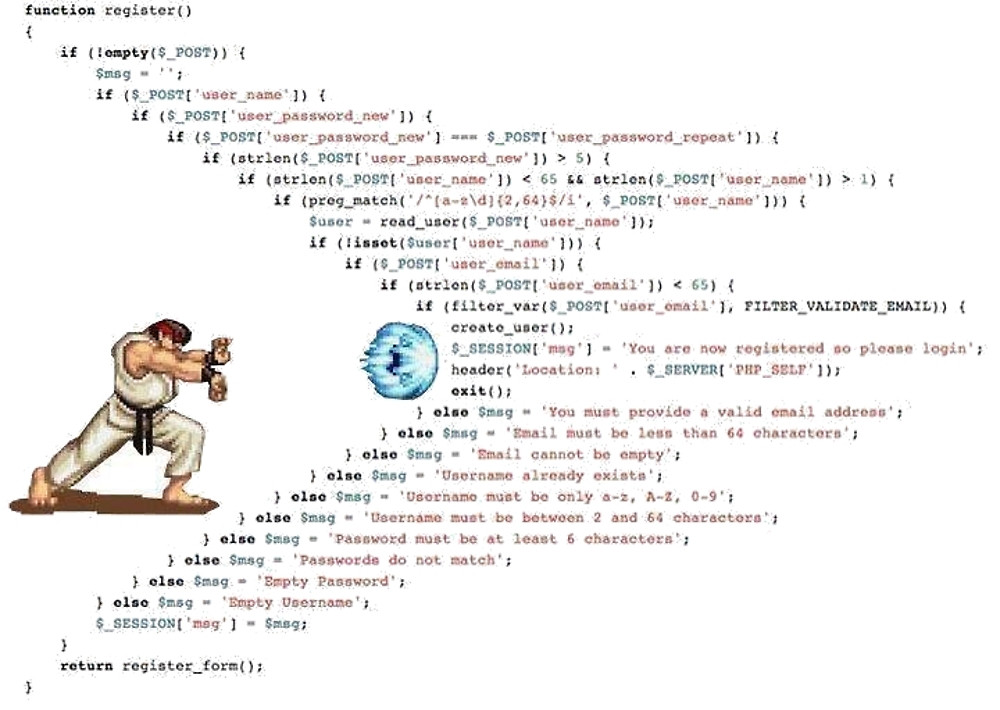# 站内搜索## 依次执行多项异步任务

``````class Point{
constructor(x, y){
this._x = x;
this._y = y;
}
get length(){
const {_x, _y} = this;
return Math.sqrt(_x * _x + _y * _y);
}
}

let p = new Point(3, 4);
console.log(p._x, p._y, p.length); //3, 4, 5
``````